## Polygon

A closed plane figure withsides. If all sides and angles are equivalent, the polygon is called regular. Regular polygons can be Convex or Star. The word derives from the Greek poly (many) and gonu (knee).

The Area of a Convex Polygon with Vertices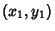, ...,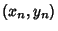is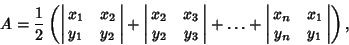(1)

which can be written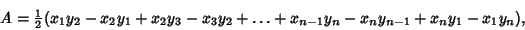(2)

where the signs can be found from the following diagram.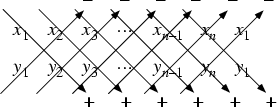The Area of a polygon is defined to be Positive if the points are arranged in a counterclockwise order, and Negative if they are in clockwise order (Beyer 1987).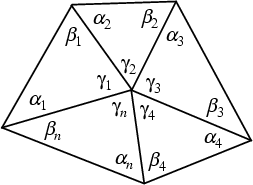The sum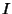of internal angles in the above diagram of a dissected polygon is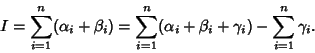(3)

But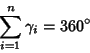(4)

and the sum of Angles of theTriangles is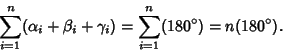(5)

Therefore,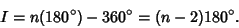(6)

Letbe the number of sides. The regular-gon is then denoted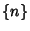.2 Digon 3 Equilateral Triangle (Trigon) 4 Square (Quadrilateral, Tetragon) 5 Pentagon 6 Hexagon 7 Heptagon 8 Octagon 9 Nonagon (Enneagon) 10 Decagon 11 Undecagon (Hendecagon) 12 Dodecagon 13 Tridecagon (Triskaidecagon) 14 Tetradecagon (Tetrakaidecagon) 15 Pentadecagon (Pentakaidecagon) 16 Hexadecagon (Hexakaidecagon) 17 Heptadecagon (Heptakaidecagon) 18 Octadecagon (Octakaidecagon) 19 Enneadecagon (Enneakaidecagon) 20 Icosagon 30 Triacontagon 40 Tetracontagon 50 Pentacontagon 60 Hexacontagon 70 Heptacontagon 80 Octacontagon 90 Enneacontagon 100 Hectogon 10000 Myriagon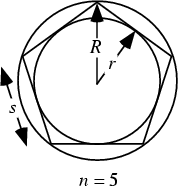Let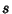be the side length,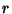be the Inradius, and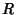the Circumradius. Then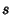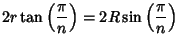(7)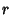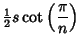(8)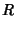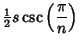(9)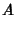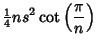(10)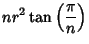(11)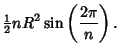(12)

If the number of sides is doubled, then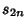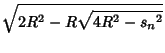(13)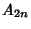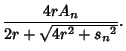(14)

Furthermore, if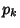and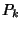are the Perimeters of the regular polygons inscribed in and circumscribed around a given Circle and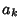and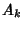their areas, then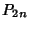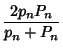(15)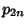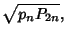(16)

and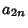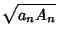(17)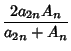(18)

(Beyer 1987, p. 125).

Compass and Straightedge constructions dating back to Euclidwere capable of inscribing regular polygons of 3, 4, 5, 6, 8, 10, 12, 16, 20, 24, 32, 40, 48, 64, ..., sides. However, this listing is not a complete enumeration of constructible'' polygons. In fact, a regular-gon is constructible only if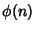is a Power of 2, where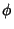is the Totient Function (this is a Necessary but not Sufficient condition). More specifically, a regular-gon (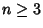) can be constructed by Straightedge and Compass (i.e., can have trigonometric functions of its Angles expressed in terms of finite Square Root extractions) Iff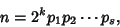(19)

where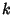is in Integer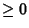and the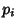are distinct Fermat Primes. Fermat Numbers are of the form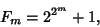(20)

whereis an Integer. The only known Primes of this form are 3, 5, 17, 257, and 65537.

The fact that this condition was Sufficient was first proved by Gaußin 1796 when he was 19 years old, and it relies on the property of Irreducible Polynomials that Roots composed of a finite number of Square Root extractions exist only if the order of the equation is of the form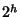. That this condition was also Necessary was not explicitly proven by Gauss, and the first proof of this fact is credited to Wantzel (1836).

Constructible values offor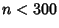were given by Gauss (Smith 1994), and the first few are 2, 3, 4, 5, 6, 8, 10, 12, 15, 16, 17, 20, 24, 30, 32, 34, 40, 48, 51, 60, 64, 68, 80, 85, 96, 102, 120, 128, 136, 160, 170, 192, ... (Sloane's A003401). Gardner (1977) and independently Watkins (Conway and Guy 1996) noticed that the number of sides for constructible polygons with an Odd number of sides is given by the first 32 rows of Pascal's Triangle (mod 2) interpreted as Binary numbers, giving 1, 3, 5, 15, 17, 51, 85, 255, ... (Sloane's A004729, Conway and Guy 1996, p. 140).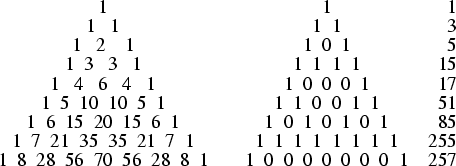Although constructions for the regular Triangle, Square, Pentagon, and their derivatives had been given by Euclid,constructions based on the Fermat Primes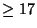were unknown to the ancients. The first explicit construction of a Heptadecagon (17-gon) was given by Erchinger in about 1800. Richelot and Schwendenwein found constructions for the 257-gon in 1832, and Hermes spent 10 years on the construction of the 65537-gon at Göttingen around 1900 (Coxeter 1969). Constructions for the Equilateral Triangle and Square are trivial (top figures below). Elegant constructions for the Pentagon and Heptadecagon are due to Richmond (1893) (bottom figures below).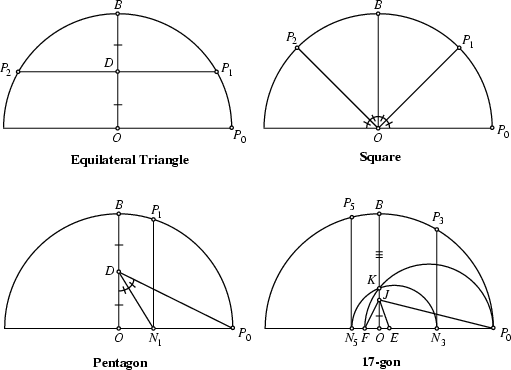Given a point, a Circle may be constructed of any desired Radius, and a Diameter drawn through the center. Call the center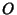, and the right end of the Diameter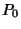. The Diameter Perpendicular to the original Diameter may be constructed by finding the Perpendicular Bisector. Call the upper endpoint of this Perpendicular Diameter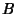. For the Pentagon, find the Midpoint of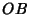and call it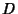. Draw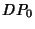, and Bisect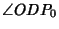, calling the intersection point with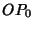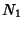. Draw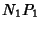Parallel to, and the first two points of the Pentagon areand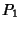. The construction for the Heptadecagon is more complicated, but can be accomplished in 17 relatively simple steps. The construction problem has now been automated (Bishop 1978).

See also 257-gon, 65537-gon, Anthropomorphic Polygon, Bicentric Polygon, Carnot's Polygon Theorem, Chaos Game, Convex Polygon, Cyclic Polygon, de Moivre Number, Diagonal (Polygon), Equilateral Triangle, Euler's Polygon Division Problem, Heptadecagon, Hexagon, Hexagram, Illumination Problem, Jordan Polygon, Lozenge, Octagon, Parallelogram, Pascal's Theorem, Pentagon, Pentagram, Petrie Polygon, Polygon Circumscribing Constant, Polygon Inscribing Constant, Polygonal Knot, Polygonal Number, Polygonal Spiral, Polygon Triangulation, Polygram, Polyhedral Formula, Polyhedron, Polytope, Quadrangle, Quadrilateral, Regular Polygon, Reuleaux Polygon, Rhombus, Rotor, Simple Polygon, Simplicity, Square, Star Polygon, Trapezium, Trapezoid, Triangle, Visibility, Voronoi Polygon, Wallace-Bolyai-Gerwein Theorem

References

Beyer, W. H. CRC Standard Mathematical Tables, 28th ed. Boca Raton, FL: CRC Press, pp. 124-125 and 196, 1987.

Bishop, W. How to Construct a Regular Polygon.'' Amer. Math. Monthly 85, 186-188, 1978.

Conway, J. H. and Guy, R. K. The Book of Numbers. New York: Springer-Verlag, pp. 140 and 197-202, 1996.

Courant, R. and Robbins, H. Regular Polygons.'' §3.2 in What is Mathematics?: An Elementary Approach to Ideas and Methods, 2nd ed. Oxford, England: Oxford University Press, pp. 122-125, 1996.

Coxeter, H. S.M. Introduction to Geometry, 2nd ed. New York: Wiley, 1969.

De Temple, D. W. Carlyle Circles and the Lemoine Simplicity of Polygonal Constructions.'' Amer. Math. Monthly 98, 97-108, 1991.

Gardner, M. Mathematical Carnival: A New Round-Up of Tantalizers and Puzzles from Scientific American. New York: Vintage Books, p. 207, 1977.

Gauss, C. F. §365 and 366 in Disquisitiones Arithmeticae. Leipzig, Germany, 1801. Translated by A. A Clarke. New Haven, CT: Yale University Press, 1965.

The Math Forum. Naming Polygons and Polyhedra.'' http://forum.swarthmore.edu/dr.math/faq/faq.polygon.names.html.

Rawles, B. Sacred Geometry Design Sourcebook: Universal Dimensional Patterns. Nevada City, CA: Elysian Pub., p. 238, 1997.

Richmond, H. W. A Construction for a Regular Polygon of Seventeen Sides.'' Quart. J. Pure Appl. Math. 26, 206-207, 1893.

Sloane, N. J. A. Sequences A004729 and A003401/M0505 in An On-Line Version of the Encyclopedia of Integer Sequences.'' http://www.research.att.com/~njas/sequences/eisonline.html and Sloane, N. J. A. and Plouffe, S. The Encyclopedia of Integer Sequences. San Diego: Academic Press, 1995.

Smith, D. E. A Source Book in Mathematics. New York: Dover, p. 350, 1994.

Tietze, H. Ch. 9 in Famous Problems of Mathematics. New York: Graylock Press, 1965.

Wantzel, M. L. Recherches sur les moyens de reconnaître si un Problème de Géométrie peut se résoudre avec la règle et le compas.'' J. Math. pures appliq. 1, 366-372, 1836.